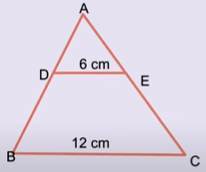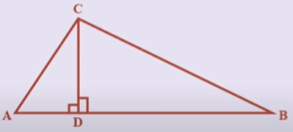Friday, October 22, 2021
Home > CBSE Class 10 > Important MCQ Questions for Class 10 Maths Chapter 6 Triangles

# Important MCQ Questions for Class 10 Maths Chapter 6 TrianglesHi students, Welcome to AMBiPi (Amans Maths Blogs). In this article, you will get Important MCQ Questions for Class 10 Maths Chapter 6 Triangles. You can download this PDF and save it in your mobile device or laptop etc.

Important MCQ Questions of Triangles Class 10 Question No 21:

If ∆ABC ~ ∆QRP and ar(∆ABC)/ar(∆PQR) = 9/4, AB = 18 cm and BC = 15 cm, then PR is equal to

Option A : 10 cm

Option B : 12 cm

Option C : 20/3 cm

Option D : 8 cm

Option A : 10 cm

Important MCQ Questions of Triangles Class 10 Question No 22:

If S is a point on PQ of a ∆PQR such that PS = QS = RS, then

Option A : PR.QR = RS2

Option B : QS2 + RS2 = QR2

Option C : PR2 + QR2 = PQ2

Option D : PS2 + RS2 = PR2

Option C : PR2 + QR2 = PQ2

Important MCQ Questions of Triangles Class 10 Question No 23:

Find the value of x in the figure below if DE || AB.Option A : x = 2

Option B : x = 1

Option C : x = 6

Option D : x = 4

Option A : x = 2

Important MCQ Questions of Triangles Class 10 Question No 24:

Diagonals of a trapezium PQRS intersect each other at the point O. PQ || RS and PQ = 3RS. Find the ratio of the areas of the triangles ∆POQ and ∆ROS.

Option A : 5 : 4

Option B : 6 : 9

Option C : 9 : 1

Option D : 1 : 2

Option C : 9 : 1

Important MCQ Questions of Triangles Class 10 Question No 25:

Find the altitude of an equilateral triangle of side 8 cm.

Option A : 2√3 cm

Option B : 3√3 cm

Option C : 4√3 cm

Option D : 5√3 cm

Option A : 13

Important MCQ Questions of Triangles Class 10 Question No 26:

If ∆ABC ~ ∆DEF, AB = 4 cm, DE = 6 cm, EF = 9 cm, FD = 12 cm. Find the perimeter of the triangle ABC.

Option A : 16 cm

Option B : 13 cm

Option C : 15 cm

Option D : 18 cm

Option D : 18 cm

Important MCQ Questions of Triangles Class 10 Question No 27:

In the figure below, if DE || BC, then the ratio of areas of the triangle ADE and the area of the quadrilateral (DECB).Option A : 1 : 2

Option B : 1 : 4

Option C : 1 : 3

Option D : 2 : 3

Option C : 1 : 3

Important MCQ Questions of Triangles Class 10 Question No 28:

Corresponding sides of two similar triangles are in the ratio of 2 : 3. If the area of smaller triangle is 48 cm2. Then, find the area of the larger triangle.

Option A : 97 cm2

Option B : 108 cm2

Option C : 107 cm2

Option D : 204 cm2

Option B : 108 cm2

Important MCQ Questions of Triangles Class 10 Question No 29:

Areas of two similar triangles are in 36cm2 and 100 cm2. If the length of a side of the larger triangle is 20 cm. Then, what is the length of the corresponding side of the smaller triangle.

Option A : 10 cm

Option B : 15 cm

Option C : 12 cm

Option D : None of these

Option C : 12 cm

Important MCQ Questions of Triangles Class 10 Question No 30:

In figure below, if the triangles ACB is similar to the triangle CDA and if AC = 8 cm and AD = 3 cm, then find the value of BD.Option A : 54/3 cm

Option B : 52/3 cm

Option C : 45/7 cm

Option D : 55/3 cm